# Thermodynamic cycle

A thermodynamic cycle consists of a linked sequence of thermodynamic processes that involve transfer of heat and work into and out of the system, while varying pressure, temperature, and other state variables within the system, and that eventually returns the system to its initial state. In the process of passing through a cycle, the working fluid (system) may convert heat from a warm source into useful work, and dispose of the remaining heat to a cold sink, thereby acting as a heat engine. Conversely, the cycle may be reversed and use work to move heat from a cold source and transfer it to a warm sink thereby acting as a heat pump.

During a closed cycle, the system returns to its original thermodynamic state of temperature and pressure. Process quantities (or path quantities), such as heat and work are process dependent. For a cycle for which the system returns to its initial state the first law of thermodynamics applies:The above states that there is no change of the energy of the system over the cycle. Ein might be the work and heat input during the cycle and Eout would be the work and heat output during the cycle. The first law of thermodynamics also dictates that the net heat input is equal to the net work output over a cycle (we account for heat, Qin, as positive and Qout as negative). The repeating nature of the process path allows for continuous operation, making the cycle an important concept in thermodynamics. Thermodynamic cycles are often represented mathematically as quasistatic processes in the modeling of the workings of an actual device.

## Heat and work

Two primary classes of thermodynamic cycles are power cycles and heat pump cycles. Power cycles are cycles which convert some heat input into a mechanical work output, while heat pump cycles transfer heat from low to high temperatures by using mechanical work as the input. Cycles composed entirely of quasistatic processes can operate as power or heat pump cycles by controlling the process direction. On a pressure-volume (PV) diagram or temperature-entropy diagram, the clockwise and counterclockwise directions indicate power and heat pump cycles, respectively.

### Relationship to work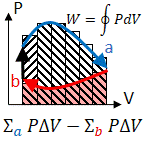The net work equals the area inside because it is (a) the Riemann sum of work done on the substance due to expansion, minus (b) the work done to re-compress.

Because the net variation in state properties during a thermodynamic cycle is zero, it forms a closed loop on a PV diagram. A PV diagram's Y axis shows pressure (P) and X axis shows volume (V). The area enclosed by the loop is the work (W) done by the process:This work is equal to the balance of heat (Q) transferred into the system:Equation (2) makes a cyclic process similar to an isothermal process: even though the internal energy changes during the course of the cyclic process, when the cyclic process finishes the system's energy is the same as the energy it had when the process began.

If the cyclic process moves clockwise around the loop, then W will be positive, and it represents a heat engine. If it moves counterclockwise, then W will be negative, and it represents a heat pump.

### Each Point in the CycleDescription of each point in the thermodynamic cycles.

Otto Cycle:

1→2: Isentropic Expansion: Constant entropy (s), Decrease in pressure (P), Increase in volume (v), Decrease in temperature (T)

2→3: Isochoric Cooling: Constant volume(v), Decrease in pressure (P), Decrease in entropy (S), Decrease in temperature (T)

3→4: Isentropic Compression: Constant entropy (s), Increase in pressure (P), Decrease in volume (v), Increase in temperature (T)

4→1: Isochoric Heating: Constant volume (v), Increase in pressure (P), Increase in entropy (S), Increase in temperature (T)

A List of Thermodynamic Processes:

Adiabatic : No energy transfer as heat (Q) during that part of the cycle would amount to δQ=0. This does not exclude energy transfer as work.

Isothermal : The process is at a constant temperature during that part of the cycle (T=constant, δT=0). This does not exclude energy transfer as heat or work.

Isobaric : Pressure in that part of the cycle will remain constant. (P=constant, δP=0). This does not exclude energy transfer as heat or work.

Isochoric : The process is constant volume (V=constant, δV=0). This does not exclude energy transfer as heat or work.

Isentropic : The process is one of constant entropy (S=constant, δS=0). This excludes the transfer of heat but not work.

### Power cycles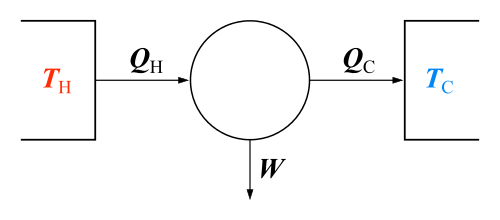Heat engine diagram.
Main article: Heat engine

Thermodynamic power cycles are the basis for the operation of heat engines, which supply most of the world's electric power and run the vast majority of motor vehicles. Power cycles can be organized into two categories: real cycles and ideal cycles. Cycles encountered in real world devices (real cycles) are difficult to analyze because of the presence of complicating effects (friction), and the absence of sufficient time for the establishment of equilibrium conditions. For the purpose of analysis and design, idealized models (ideal cycles) are created; these ideal models allow engineers to study the effects of major parameters that dominate the cycle without having to spend significant time working out intricate details present in the real cycle model.

Power cycles can also be divided according to the type of heat engine they seek to model. The most common cycles used to model internal combustion engines are the Otto cycle, which models gasoline engines, and the Diesel cycle, which models diesel engines. Cycles that model external combustion engines include the Brayton cycle, which models gas turbines, the Rankine cycle, which models steam turbines, the Stirling cycle, which models hot air engines, and the Ericsson cycle, which also models hot air engines.The clockwise thermodynamic cycle indicated by the arrows shows that the cycle represents a heat engine. The cycle consists of four states (the point shown by crosses) and four thermodynamic processes (lines).

For example, the pressure-volume mechanical work output from the heat engine cycle (net work out), consisting of 4 thermodynamic processes, is:If no volume change happens in process 4-1 and 2-3, equation (3) simplifies to:### Heat pump cycles

Thermodynamic heat pump cycles are the models for household heat pumps and refrigerators. There is no difference between the two except the purpose of the refrigerator is to cool a very small space while the household heat pump is intended to warm a house. Both work by moving heat from a cold space to a warm space. The most common refrigeration cycle is the vapor compression cycle, which models systems using refrigerants that change phase. The absorption refrigeration cycle is an alternative that absorbs the refrigerant in a liquid solution rather than evaporating it. Gas refrigeration cycles include the reversed Brayton cycle and the Hampson-Linde cycle. Multiple compression and expansion cycles allow gas refrigeration systems to liquify gases.

## Modelling real systems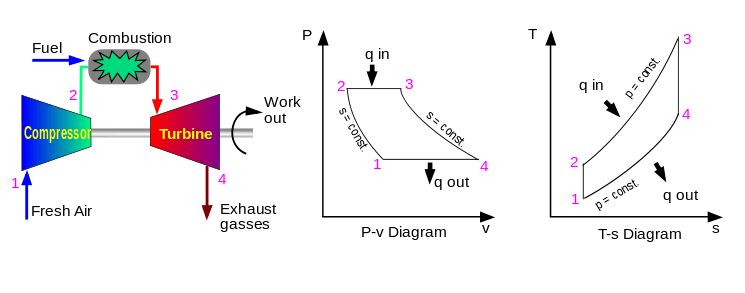Example of a real system modelled by an idealized process: PV and TS diagrams of a Brayton cycle mapped to actual processes of a gas turbine engine

Thermodynamic cycles may be used to model real devices and systems, typically by making a series of assumptions. simplifying assumptions are often necessary to reduce the problem to a more manageable form. For example, as shown in the figure, devices such a gas turbine or jet engine can be modeled as a Brayton cycle. The actual device is made up of a series of stages, each of which is itself modeled as an idealized thermodynamic process. Although each stage which acts on the working fluid is a complex real device, they may be modelled as idealized processes which approximate their real behavior. If energy is added by means other than combustion, then a further assumption is that the exhaust gases would be passed from the exhaust to a heat exchanger that would sink the waste heat to the environment and the working gas would be reused at the inlet stage.

The difference between an idealized cycle and actual performance may be significant. For example, the following images illustrate the differences in work output predicted by an ideal Stirling cycle and the actual performance of a Stirling engine: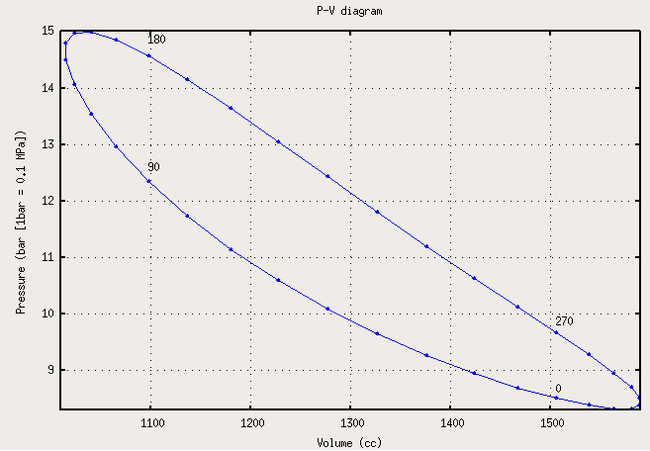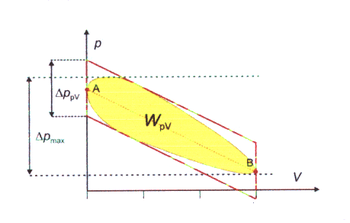Ideal Stirling cycle Actual performance Actual and ideal overlaid, showing difference in work output

As the net work output for a cycle is represented by the interior of the cycle, there is a significant difference between the predicted work output of the ideal cycle and the actual work output shown by a real engine. It may also be observed that the real individual processes diverge from their idealized counterparts; e.g., isochoric expansion (process 1-2) occurs with some actual volume change.

## Well-known thermodynamic cycles

In practice, simple idealized thermodynamic cycles are usually made out of four thermodynamic processes. Any thermodynamic processes may be used. However, when idealized cycles are modeled, often processes where one state variable is kept constant are used, such as an isothermal process (constant temperature), isobaric process (constant pressure), isochoric process (constant volume), isentropic process (constant entropy), or an isenthalpic process (constant enthalpy). Often adiabatic processes are also used, where no heat is exchanged.

Some example thermodynamic cycles and their constituent processes are as follows:

Cycle Process 1-2
(Compression)
Process 2-3
Process 3-4
(Expansion)
Process 4-1
(Heat Rejection)
Notes
Power cycles normally with external combustion - or heat pump cycles:
Carnot isentropic isothermal isentropic isothermal Carnot heat engine
Ericsson isothermal isobaric isothermal isobaric the second Ericsson cycle from 1853
and volume
Stirling isothermal isochoric isothermal isochoric Stirling engine
Manson isothermal isochoric isothermal isochoric then adiabatic Manson-Guise Engine
Power cycles normally with internal combustion:
the external combustion version of this cycle is known as first Ericsson cycle from 1833
Lenoir isobaric isochoric adiabatic Pulse jets
(Note: Process 1-2 accomplishes both the heat rejection and the compression)

### Ideal cycle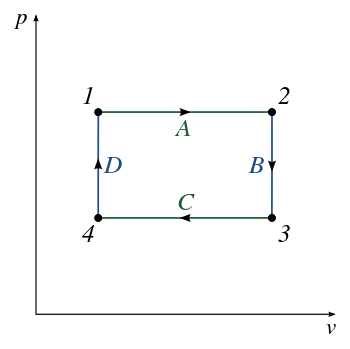An illustration of an ideal cycle heat engine (arrows clockwise).

An ideal cycle is constructed out of:

1. TOP and BOTTOM of the loop: a pair of parallel isobaric processes
2. LEFT and RIGHT of the loop: a pair of parallel isochoric processes

Internal energy of a perfect gas undergoing different portions of a cycle:

Isothermal:Isochoric:Isobaric:### Carnot cycle

Main article: Carnot cycle

The Carnot cycle is a cycle composed of the totally reversible processes of isentropic compression and expansion and isothermal heat addition and rejection. The thermal efficiency of a Carnot cycle depends only on the absolute temperatures of the two reservoirs in which heat transfer takes place, and for a power cycle is:whereis the lowest cycle temperature andthe highest. For Carnot power cycles the coefficient of performance for a heat pump is:and for a refrigerator the coefficient of performance is:The second law of thermodynamics limits the efficiency and COP for all cyclic devices to levels at or below the Carnot efficiency. The Stirling cycle and Ericsson cycle are two other reversible cycles that use regeneration to obtain isothermal heat transfer.

### Stirling cycle

Main article: Stirling cycle

A Stirling cycle is like an Otto cycle, except that the adiabats are replaced by isotherms. It is also the same as an Ericsson cycle with the isobaric processes substituted for constant volume processes.

1. TOP and BOTTOM of the loop: a pair of quasi-parallel isothermal processes
2. LEFT and RIGHT sides of the loop: a pair of parallel isochoric processes

Heat flows into the loop through the top isotherm and the left isochore, and some of this heat flows back out through the bottom isotherm and the right isochore, but most of the heat flow is through the pair of isotherms. This makes sense since all the work done by the cycle is done by the pair of isothermal processes, which are described by Q=W. This suggests that all the net heat comes in through the top isotherm. In fact, all of the heat which comes in through the left isochore comes out through the right isochore: since the top isotherm is all at the same warmer temperatureand the bottom isotherm is all at the same cooler temperature, and since change in energy for an isochore is proportional to change in temperature, then all of the heat coming in through the left isochore is cancelled out exactly by the heat going out the right isochore.

## State functions and entropy

If Z is a state function then the balance of Z remains unchanged during a cyclic process:.

Entropy is a state function and is defined asso that,

then it is clear that for any cyclic process,meaning that the net entropy change over a cycle is 0.Wikimedia Commons has media related to Thermodynamics cycles.